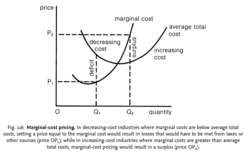# marginal-cost pricing

Also found in: Dictionary, Thesaurus, Medical, Encyclopedia.

## marginal-cost pricing

see COST BASED PRICING.
Collins Dictionary of Business, 3rd ed. © 2002, 2005 C Pass, B Lowes, A Pendleton, L Chadwick, D O’Reilly and M AffersonFig. 116 Marginal-cost pricing. In decreasing-cost industries where marginal costs are below average total costs, setting a price equal to the marginal cost would result in losses that would have to be met from taxes or other sources (price OP1 ); while in increasing-cost industries where marginal costs are greater than average total costs, marginal-cost pricing would result in a surplus (price OP2 ).

## marginal-cost pricing

the setting of a PRICE for a product that is based upon the MARGINAL COST of producing and distributing it.
1. a pricing method that sets the price for a product based upon the CONTRIBUTION (i.e. price less unit VARIABLE COST or marginal cost) needed for the product to BREAK EVEN or provide a predetermined target level of PROFIT. Unit contribution when multiplied by sales/production volume gives total contribution, and this contribution should provide sufficient money to cover FIXED COSTS (to break even) or fixed costs and target profit. To calculate selling price, it is necessary to add unit contribution to unit variable cost or marginal cost. Although this pricing method is based upon costs, in practice managers take into account demand and competition by varying the target unit contribution. Compare FULL-COST PRICING.
2. a pricing principle that argues for setting prices equal to the marginal cost of production and distribution, ignoring whether or not fixed costs are recouped from revenues. The principle has been advocated as a guide to the pricing and output policies of PUBLIC UTILITIES on the grounds that prices that reflect the marginal cost to society of producing an extra unit of output are more socially desirable. The principle is particularly appealing when used for public utilities where marginal costs are effectively zero, such as parks, bridges and museums, because here society can be made better off by lowering the price of these facilities until they are fully used. The marginal-cost pricing principle however, deals only with the short-run problem of generating an optimum price and output with existing capacity and ignores the long-run problem of ideal investment in new capacity. See also AVERAGE-COST PRICING, TWO-PART TARIFF.
Collins Dictionary of Economics, 4th ed. © C. Pass, B. Lowes, L. Davies 2005
References in periodicals archive ?
The basic conceptual argument for the efficiency of marginal-cost pricing is well-known (see, for example, Baumol and Bradford 1970).
(which would also imply that, [[Epsilon].sub.A] = [infinity], or that the demand for ancillary coverages is perfectly elastic) would lead to marginal-cost pricing. However, such perfectly elastic demand curves are not permissible in this model.
(This can be compared to the price Pc and quantity QC that would be yielded by a marginal-cost pricing regime.) The monopolist's markup over marginal costs is the vertical distance between PM and MC.
The practice of marginal-cost pricing can be attributed to the particular roles of entrance fees and per-unit prices in profit functions.

Site: Follow: Share:
Open / Close